# Making a Slow Pad with the Malstrm

### Making a Slow Pad with the Malström

Now let's use some of what we've learned to create a slow, evolving pad with just one note in our Sequencer.

Here's an example of a creepy, evolving pad that uses one modulator on each oscillator with different shapes and very low rates. This sound uses no filtering or tone shaping. When the filters aren't being used, you can bypass the master controls altogether and run sound out the oscillator outputs instead of the default main outputs.

First we'll set up Osc A to play a deep, low-frequency pad.

#### To set up and program Osc A

1.

2.

Make a new eight-bar loop with just one four-bar note at G3 (Figure 5.101), and press play.

##### Figure 5.101. Sequencer set up for a long, evolving pad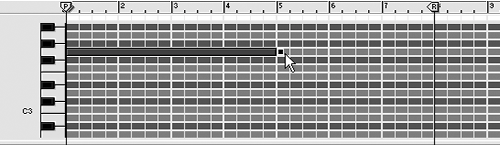3.

At the far-right of the Malström, move the Spread knob hard right so each oscillator is coming out of a separate channel.

4.

We're going to set up Osc A to be a slow metallic pad. Set Osc A to the Voice:TibetanMonks waveform.

5.

Set the Index to 57 and the Motion to 22 (Figure 5.102).

##### Figure 5.102. Change the index and motion for this Osc A pad.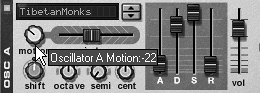6.

To deepen the voice, set the Octave knob to 3 and the Shift knob down to 24 (Figure 5.103).

##### Figure 5.103. Pick a lower octave and a lower formant setting for Osc A.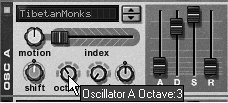7.

For infinite sustain, set the Osc A release fader to 127 (Figure 5.104).

##### Figure 5.104. Set the Osc A release time for infinity.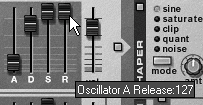Now let's set up a slow-moving wave to modulate the tone of Osc A.

#### To set up Mod A to control Osc A

1.

Enable Mod A and set the destination switch to A (Osc A).

2.

Turn on Sync for Mod A, and select curve 7 (Figure 5.105).

##### Figure 5.105. Select curve 7 for Mod A.3.

Slow the Mod A Rate hard left to 16/4, and the Mod A Index knob left to 26 (Figure 5.106).

##### Figure 5.106. Change the Mod A Index and Motion settings.4.

Turn the Mod A Shift knob right to 40 (Figure 5.107).

##### Figure 5.107. Increase Mod A's Shift.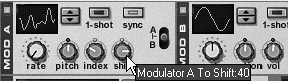We'll use a bottle sound in Osc B to float against Osc A.

#### To set up Osc B

1.

Enable Osc B and select the Wind:Bottle waveform.

2.

Set the Motion knob left to 44 and the Index to 43 (Figure 5.108).

##### Figure 5.108. Increase the Osc B Index and decrease Motion.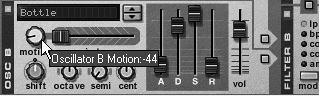3.

Set the Shift knob to 10 and turn the Octave knob left to 2 (Figure 5.109).

##### Figure 5.109. Set Osc B shift to 10 and Index to 2.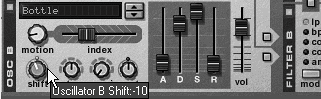4.

Set the Osc B attack and release sliders to 87 (Figure 5.110).

##### Figure 5.110. Increase attack and release for the Osc B envelope.Now we'll set up a regular, sine-shaped modulation curve for Osc B.

#### To modulate Osc B and Mod A

1.

Enable Mod B, turn on Sync (Figure 5.111), and set the destination to B (Osc B).

##### Figure 5.111. Enable Sync for Mod B.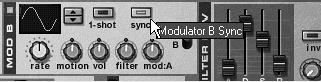2.

Set the Mod B Rate to 16/4 and the Mod B Motion knob to 38.

3.

Set the Mod B Mod:A knob hard right to 63 (Figure 5.112).

##### Figure 5.112. Set Mod B's Mod A control all the way right.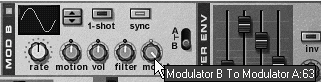Reason 3 For Windows and Mac
ISBN: 321269179
EAN: N/A
Year: 2003
Pages: 180